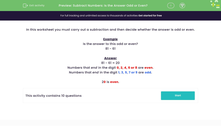# Subtract Numbers: Is the Answer Odd or Even?

In this worksheet, students carry out a subtraction and decide whether the difference is odd or even.Key stage:  KS 1

Curriculum topic:   Number: Addition and Subtraction

Curriculum subtopic:   Solve Add/Subtract Problems to 100

Popular topics:   Subtraction worksheets

Difficulty level:#### Worksheet Overview

In this worksheet you must carry out a subtraction and then decide whether the answer is odd or even.

Example

Is the answer to this odd or even?

81 - 61

81 - 61 = 20

Numbers that end in the digit 0, 2, 4, 6 or 8 are even.

Numbers that end in the digit 1, 3, 5, 7 or 9 are odd.

20 is even.

### What is EdPlace?

We're your National Curriculum aligned online education content provider helping each child succeed in English, maths and science from year 1 to GCSE. With an EdPlace account you’ll be able to track and measure progress, helping each child achieve their best. We build confidence and attainment by personalising each child’s learning at a level that suits them.

Get started